Courses

# Lakhmir Singh & Manjit Kaur: Magnetic Effects of Electric Current, Solutions- 3 Class 10 Notes | EduRev

## Class 10 : Lakhmir Singh & Manjit Kaur: Magnetic Effects of Electric Current, Solutions- 3 Class 10 Notes | EduRev

The document Lakhmir Singh & Manjit Kaur: Magnetic Effects of Electric Current, Solutions- 3 Class 10 Notes | EduRev is a part of the Class 10 Course Lakhmir Singh & Manjit Kaur Solutions: Class 10 Science.
All you need of Class 10 at this link: Class 10

Page No:92

Question 18: State Fleming’s left-hand rule. Explain it with the help of labelled diagrams.

Solution : Fleming’s left hand rule: Hold the forefinger, the centre finger and the thumb of your left hand to right angles to one other. Adjust your hand in such a way that the forefinger points in the direction of magnetic field and the and the centre finger points in the direction of current, than the direction in which thumb points, gives the direction of force acting on the conductor.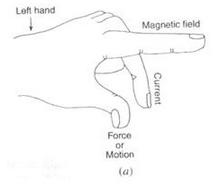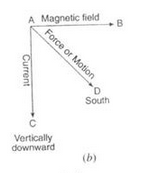Question 19: What is the principle of an electric motor ? Name some of the devices in which electric motors are used.

Solution : Fleming’s left hand rule: Hold the forefinger, the centre finger and the thumb of your left hand to right angles to one other. Adjust your hand in such a way that the forefinger points in the direction of magnetic field and the and the centre finger points in the direction of current, than the direction in which thumb points, gives the direction of force acting on the conductor.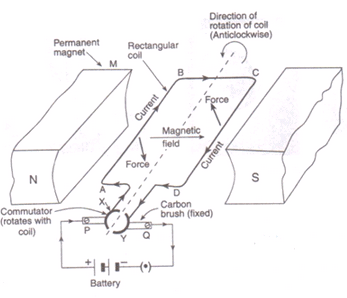Question 20: (a) In a d.c. motor, why must the current to the coil be reversed twice during each rotation ?
(b) What device reverses the current ?

Solution : (a) The current to the coil must be reversed twice during each rotation so that the coil keeps rotating continuously in the same direction.
(b) Commutator.

Question 21: (a) State what would happen to the direction of rotation of a motor if :
(i) the current were reversed
(ii) the magnetic field were reversed
(iii) both current and magnetic field were reversed simultaneously.
(b) In what ways can a motor be made more powerful ?

Solution :(a) (i) Direction of rotation would be reversed
(ii) Direction of rotation would be reversed
(iii) Direction of the rotation would remain unchanged
(b) Motor can be made more powerful by winding the coil on a soft iron core of by increasing the number of turns of the coil.

Question 22: (a) What is an electric motor ? With the help of a labelled diagram, describe the working of a simple electric motor.
(b) What are the special features of commercial electric motors ?

Solution : (a) Electric motor is a device used for converting electrical energy into mechanical energy.
Working of an electric motor: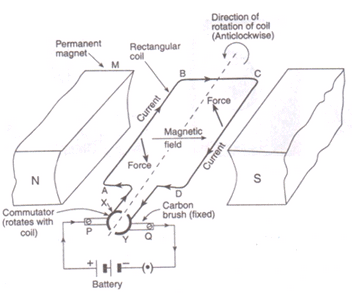Initially, the coil ABCD is in the horizontal position. On pressing the switch, current enters the coil through carbon brush P and commutator half ring X. The current flows in the direction ABCD and leaves via ring Y and brush Q. The direction of magnetic field is from N pole to S pole of the magnet. According to Fleming’s left-hand rule, the force on sides AB and CD is in the downward and upward directions respectively. This makes the coil ABCD move in the anticlockwise direction.
When the coil reaches vertical position, then the brushes P and Q will touch the gap between the two commutator rings and current is cut off. But the coil does not stop rotating as it has already gained momentum. When the coil goes beyond the vertical position, the side CD comes on the left side and side AB comes to the right side, and the two commutator rings change contact from one brush to the other. This reverses the direction of current in the coil, which in turn reverses the direction of forces acting on the sides AB and CD of the coil. The side CD is pushed down and side AB is pushed up. Thus, the coil rotates anticlockwise by another half rotation.
The reversing of current in the coil is repeated after every half rotation due to which the coil (and its shaft) continues to rotate as long as current from the battery is passed through it. The rotating shaft of electric motor can drive a large number of machines which are connected to it.
(b) In commercial electric motors:
i. the coil is wound on a soft iron core. This increases the strength of magnetic field, which makes the motor more powerful.
ii. the coil contains a large number of turns of insulated copper wire.
iii. a powerful electromagnet is used in place of permanent magnet.

Page No:93

Question 31: In the simple electric motor of figure given below, the coil rotates anticlockwise as seen by the eye from the position X when current flows in the coil. Is the current flowing clockwise or anticlockwise around the coil when viewed from above ?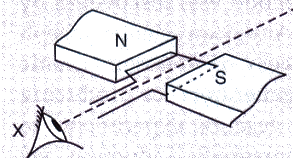Solution : Clockwise direction (according to Fleming’s left hand rule).

Question 32: Which way does the wire in the diagram below tend to move ?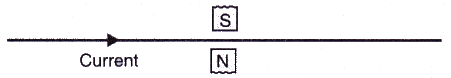Solution : According to Fleming’s left hand rule, the wire moves in the upward direction (out of the page).

Question 33: If the current in a wire is flowing in the vertically downward direction and a magnetic field is applied from west to east, what is the direction of force on the wire ?

Solution : Force will be due South (according to Fleming’s left hand rule).

Question 34: Which way does the wire in the diagram below tend to move ?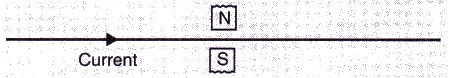Solution : According to Fleming’s left hand rule, the wire moves in the downward direction (into the page).

Question 35: What is the force on a current-carrying wire that is parallel to a magnetic field ? Give reason for your answer.

Solution : Force on a current-carrying wire that is parallel to magnetic field will be zero.
This is because the magnitude of force depends on the sin of the angle between the direction of current and the direction of magnetic field, so if the current carrying wire is held parallel to the magnetic field, the force will be zero.

Question 36: A charged particle enters at right angles into a uniform magnetic field as shown :
What should be the nature of charge on the particle if it begins to move in a direction pointing vertically out of the page due to its interaction with the magnetic field ?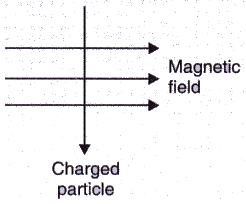Solution : Positive charge.

Page No:102

Question 1: Name the device which converts mechanical energy into electric energy.

Solution : Electric Generator.

Question 2: Out of an A.C. generator and a D.C. generator :
(a) which one uses a commutator (split rings) ?
(b) which one uses slip rings ?

Solution : (a) D.C. Generator. (b) A.C. Generator.

Question 3: Name the phenomenon which is made use of in an electric generator.

Solution : Electromagnetic Induction.

Question 4: Name the rule which gives the direction of induced current.

Solution : Fleming’s Right Hand Rule.

Question 5: What condition is necessary for the production of current by electromagnetic induction ?

Solution : The condition necessary for the production of current by electromagnetic induction is that there must be a relative motion between the wire and the magnet.

Question 6: What type of generator is used at Power Stations ?

Solution : AC Generator (or Alternator).

Question 7: What change should be made in an a.c. generator so that it may become a d.c. generator ?

Solution : If we replace the slip rings of an AC generator by a commutator, then it will become a DC generator.

Question 8: State whether the following statements are true or false :
(a) A generator works on the principle of electromagnetic induction.
(b) A motor works on the principle of electromagnetic induction.

Solution : (a) True (b) False

Question 9: What is the function of brushes in an electric generator ?

Solution : Function of brushes is to transfer the current from coil to load.

Question 10: When a wire is moved up and down in a magnetic field, a current is induced in the wire. What is this phenomenon known as ?

Solution : This phenomena is known as electromagnetic induction.

Question 11: When current is ‘switched on’ and ‘switched off’ in a coil, a current is induced in another coil kept near it. What is this phenomenon known as ?

Solution : Electromagnetic induction

Question 12: What is the major difference between the simple alternator and most practical alternators ?

Solution : Simple alternator:- Magnet fixed and coil rotates;
Practical alternator:- Coil fixed and magnet rotates.

Question 13: Why are Thermal Power Stations usually located near a river ?

Solution : To obtain water for making steam for turning turbines and for cooling spent steam to condense it back into hot water for making fresh steam.

Question 14: List three sources of magnetic fields.

Solution : Electromagnet, permanent magnet, wire carrying current.

Question 15: Complete the following sentence :
A generator with commutator produces………………… current.

Solution : direct.

Question 16: Two circular coils A and B are placed close to each other. If the current in coil A is changed, will some current be induced in the coil B ? Give reason for your answer.

Solution : Yes, some current will be induced in the coil B because of change in magnetic field through the coil B due to change in current in coil A. This is called electromagnetic induction.

Question 17: (a) Explain the principle of an electric generator.
(b) State two ways in which the current induced in the coil of a generator could be increased.

Solution : (a) Electric generator is based on the principle that when a straight conductor is moved in a magnetic field, then current is induced in the conductor.
(b) Two ways in which the current induced in the coil of a generator could be increased are:
(i) by roating the coil faster.
(ii) by using a coil with a larger area.

Question 18: (a) What is the difference between alternating current and direct current ?
(b) What type of current is given by (i) a dry cell, and (ii) a Power House generator ?

Solution : (a) The difference between AC and DC is that DC flows in one direction only while AC reverses direction after equal intervals of time.
(i) DC current remains same with time in its value and direction.
(ii) AC current changes with time and changes its direction every time after a certain interval of time.
(b)
(i) DC
(ii) AC

Question 19: State and explain Fleming’s right hand rule.

Solution : The direction of induced current produced in a straight conductor moving in a magnetic field is given by Fleming’s right hand rule.
According to Fleming’s right hand rule : Hold the thumb, the fore finger and the centre finger of your right-hand at right angles to one another. Adjust your hand in such a way that forefinger points in the direction of magnetic field, and thumb points in the direction of motion of conductor, then the direction in which centre finger points, gives the direction of induced current in the conductor.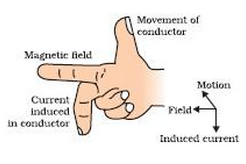Question 20: Name and state the rule to find the direction of :
current induced in a coil due to its rotation in a magnetic field.
force experienced by a current-carrying straight conductor placed in a magnetic field which is perpendicular to it.

Solution : (a) Fleming’s right hand rule:- Hold the thumb, the fore finger and the centre finger of your right-hand at right angles to one another. Adjust your hand in such a way that forefinger points in the direction of magnetic field, and thumb points in the direction of motion of conductor, then the direction in which centre finger points, gives the direction of induced current in the conductor.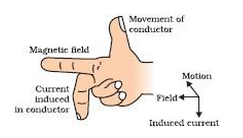(b) Fleming’s left hand rule:- Hold the forefinger, the centre finger and the thumb of your left hand at right angle to one another. Adjust your hand in such a way that the forefinger points in the direction of magnetic field and the and centre finger points in the direction of current, then the direction in which thumb points, gives the direction of force acting on the conductor.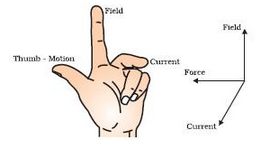Question 21: (a) In what respect does the construction of an A.C. generator differ from that of a D.C. generator ?
(b) What normally drives the alternators in a Thermal Power Station ? What fuels can be used to heat water in the boiler ?

Solution : (a). Construction-wise, the only difference between a D.C. generator and an A.C. generator is in the way the two ends of the generator coil are linked to the outer circuit. In a D.C. generator we connect the two ends of the coil to a commutator consisting of two half rings of copper. In an A.C. generator we connect the two ends of the coil to two full rings of copper called slip rings. There is no commutator in an A.C. generator.
(b) Generally, the alternators in a Thermal Power Station are driven by the power of high pressure steam.
To heat water in the boiler, fuels like coal or natural gas can be used.

Question 22: Draw the labelled diagram of an A.C. generator. With the help of this diagram, explain the construction and working of an A.C. generator.

Solution :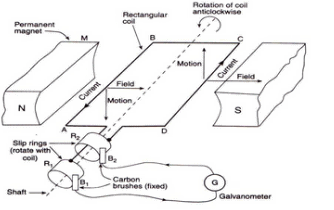Construction: A simple AC generator consists of a rectangular coil ABCD which can be rotated rapidly between the poles N and Sofa  strong horseshoe-type permanent magnet M. The coil is made of a large number of turns of insulated copper wire. The two ends A and D of the coil are connected to two circular pieces of copper metal called slip rings R1 and R2. As the slip rings rotate with the coil, the two fixed pieces of carbon called brushes, B1 and B2. keep contact with them. So. the current produced in the rotating coil can be tapped out through slip rings into the carbon brushes. The outer ends of carbon brushes are connected to a galvanometer to show the flow of current in the external circuit. Working:

Suppose the coil ABC D. which is initially in the horizontal position, is rotated in the anticlockwise direction. The side AB of the coil moves down and side CD moves up. Due to this, induced current is produced in both the sides, which flows in the direction BADC (according to Fleming's right hand rule). Thus. in the first half rotation. the current in the external circuit flows from brush B1 to B2. After half revolution. sides AB and CD will interchange their positions. So. side AB starts moving up and side CD starts moving down. As a result. direction of induced current in the coil is reversed and flows in the direction CDAB. The current in the external circuit flows from brush B2 to B1

Question 23: (a) What do you understand by the term “electromagnetic induction”? Explain with the help of a diagram.
(b) Name one device which works on the phenomenon of electromagnetic induction.
(c) Describe different ways to induce current in a coil of wire.

Solution : (a) The production of electricity from magnetism is known as electromagnetic induction.
Let us move a wire AB upward rapidly between the poles of the horseshoe magnet. When the wire is moved up, there is a deflection in the galvanometer pointer which shows a current is produced in the wire AB momentarily. Thus, as the wire is moved up through the magnetic field, an electric current is produced in it.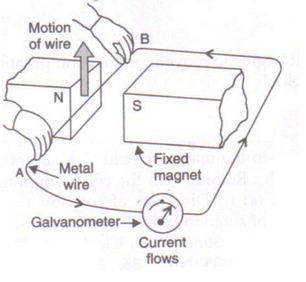(b) Electric generator.
(c) Different ways to induce current in a coil of wire are:
(i) by moving the coil relative to a fixed magnet
(ii) by keeping the coil fixed and moving a magnet relative to it.

Question 24: (a) What do you understand by the terms ‘direct current’ and ‘alternating current’ ?
(b) Name some sources of direct current and some of alternating current.
(c) State an important advantage of alternating current over direct current.
(d) What is the frequency of A.C. supply in India ?

Solution : (a) If the current flows in one direction only, it is known as direct current; and if the current reverses direction after equal intervals of time, it is called alternating current.
(b) Source of DC are dry cell, car battery, DC generator etc.
Source of AC are AC generator, bicycle dynamos etc.
(c) An important advantage of AC over DC is that AC can be transmitted over long distances without much loss of electrical energy.
(d) 50Hz.

Offer running on EduRev: Apply code STAYHOME200 to get INR 200 off on our premium plan EduRev Infinity!

61 docs

,

,

,

,

,

,

,

,

,

,

,

,

,

,

,

,

,

,

,

,

,

,

,

,

;